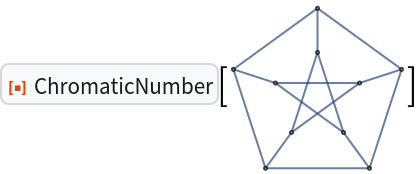Function Repository Resource:

ChromaticNumber

Compute the vertex chromatic number of a graph

Contributed by: Szabolcs Horvát and Michael Sollami
 ResourceFunction["ChromaticNumber"][g] computes the vertex chromatic number χ(g) of the simple graph g.

Details and Options

ResourceFunction["ChromaticNumber"] works on both connected and unconnected simple graphs, i.e. undirected graphs containing no self-loops or multiedges.
The chromatic number of a graph is the smallest number of colors needed to color the vertices so that no two adjacent vertices share the same color.
This function uses a linear programming based algorithm. It works well in general, but if you need faster performance, check out IGChromaticNumber and IGMinimumVertexColoring from the igraph interface IGraph/M.

Examples

Basic Examples (3)

Compute chromatic numbers of simple graphs:

 In:=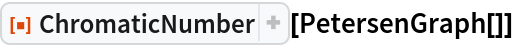Out=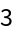Compute chromatic numbers of graphs:

 In:=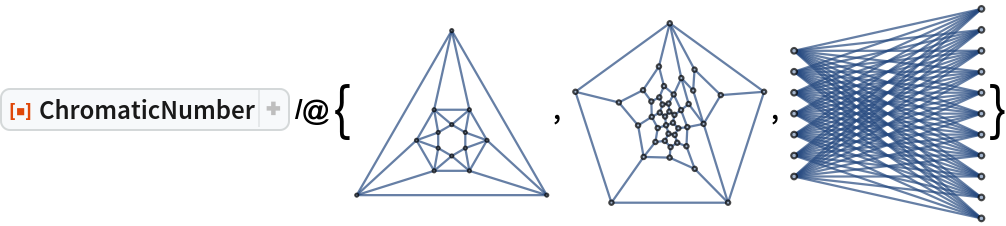Out=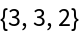Compute the vertex chromatic number of famous graphs:

 In:=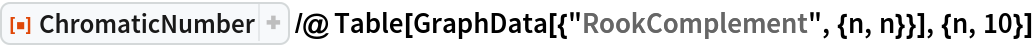Out=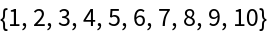Scope (2)

Works on graphs in either 2D or 3D form:

 In:=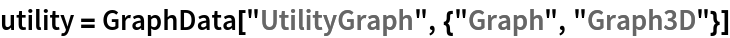Out=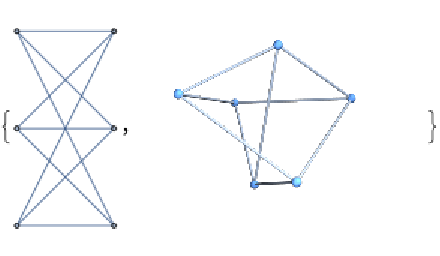In:=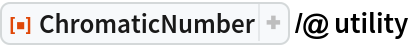Out=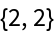Special and corner cases are handled efficiently:

 In:=Out=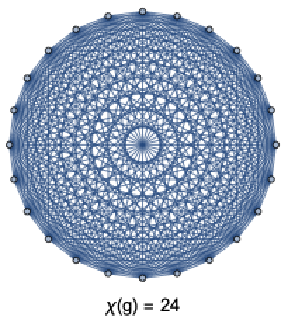Applications (1)

Compute on larger graphs than was possible before (with Combinatorica`):

 In:=Out=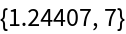Possible Issues (1)

ChromaticNumber does not work on the output of GraphPlot:

 In:=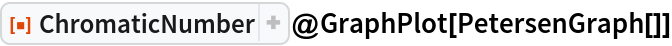Out=